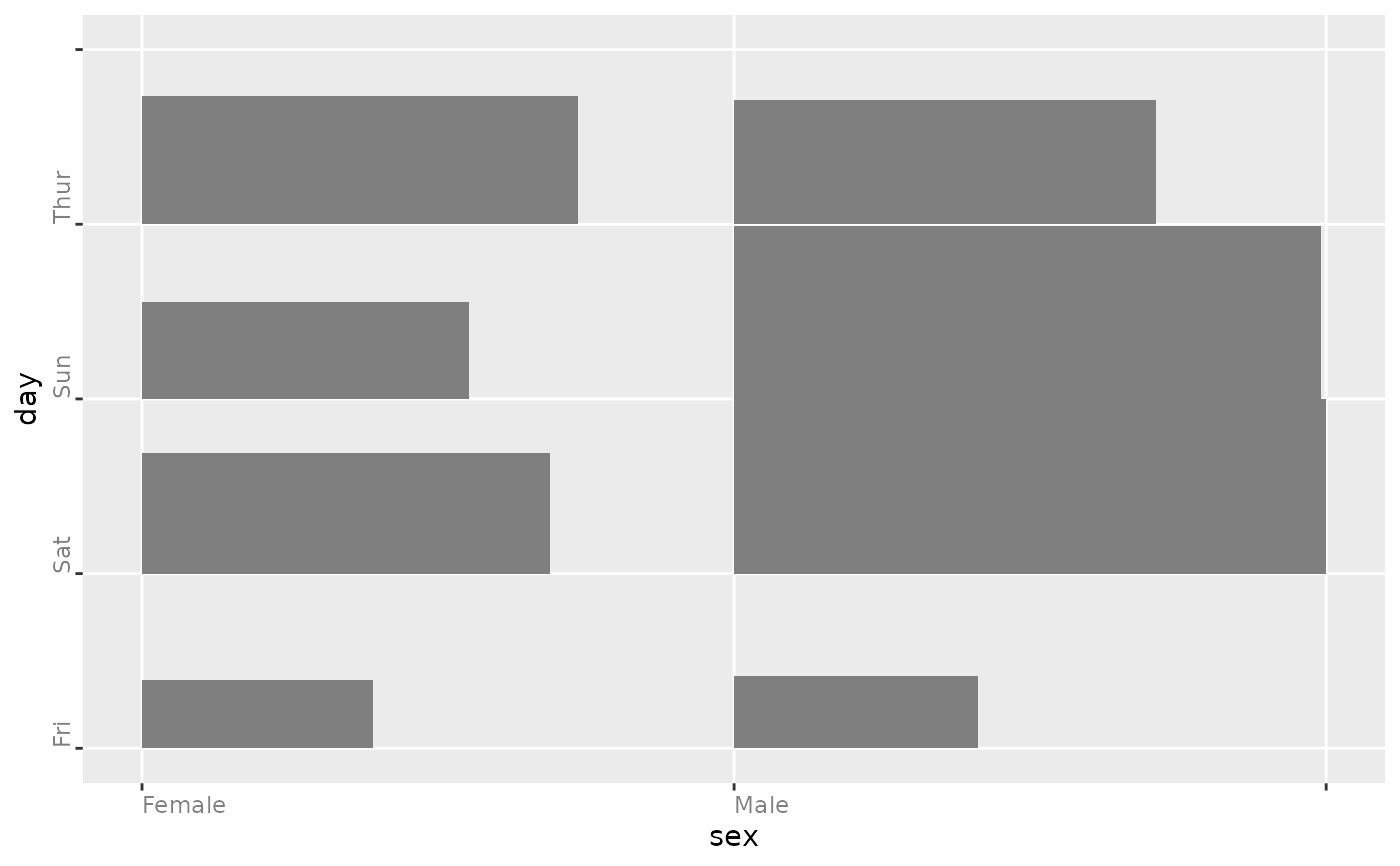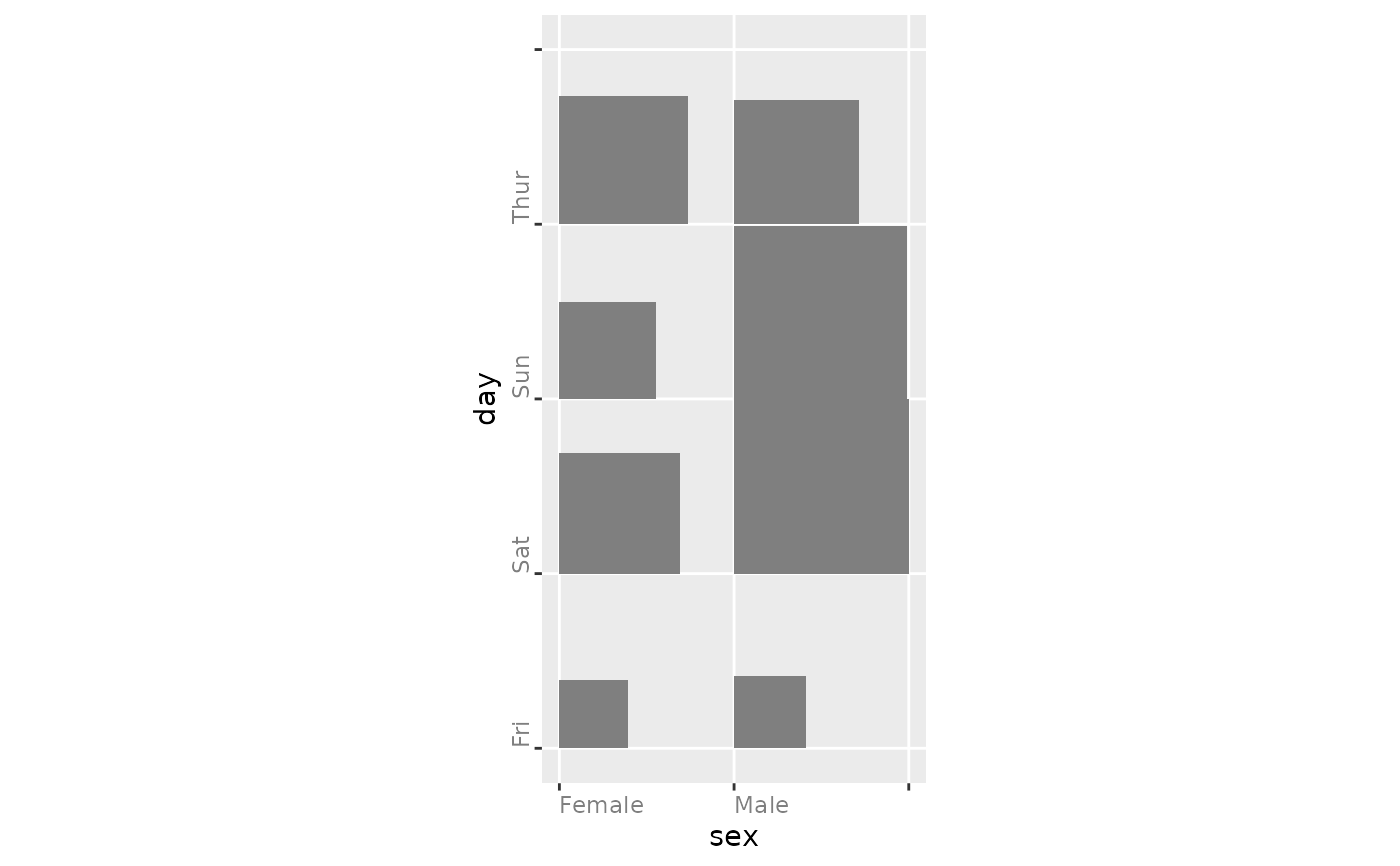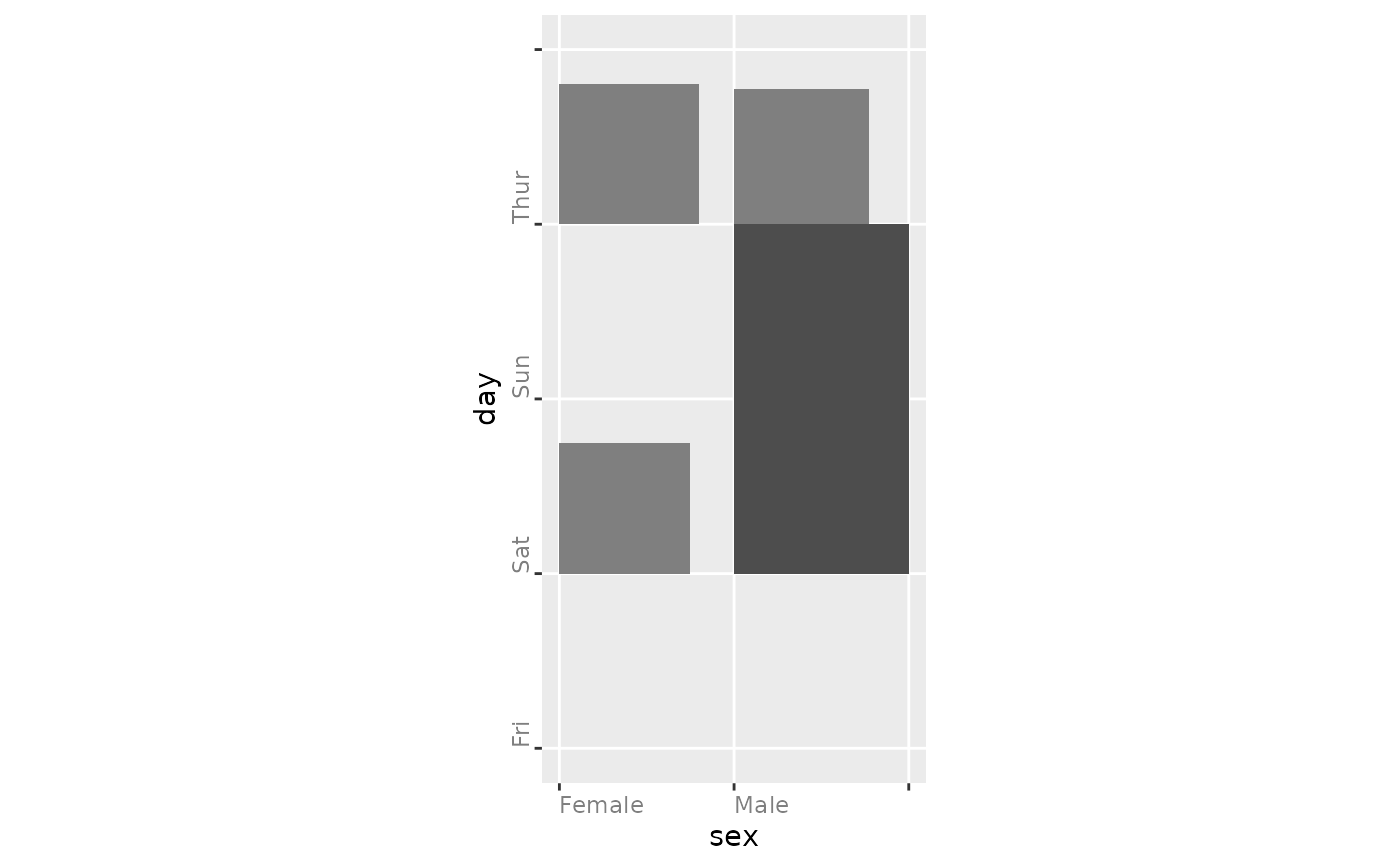Plots the mosaic plot by using fluctuation.

ggally_ratio(
data,
mapping = do.call(ggplot2::aes_string, as.list(colnames(data)[1:2])),
...,
floor = 0,
ceiling = NULL
)

## Arguments

data data set using aesthetics being used. Only x and y will used and both are required passed to geom_tile(...) don't display cells smaller than this value max value to scale frequencies. If any frequency is larger than the ceiling, the fill color is displayed darker than other rectangles

Barret Schloerke

## Examples

# Small function to display plots only if it's interactive
p_ <- GGally::print_if_interactive

data(tips, package = "reshape")
p_(ggally_ratio(tips, ggplot2::aes(sex, day)))p_(ggally_ratio(tips, ggplot2::aes(sex, day)) + ggplot2::coord_equal())# only plot tiles greater or equal to 20 and scale to a max of 50
p_(ggally_ratio(
tips, ggplot2::aes(sex, day),
floor = 20, ceiling = 50
) + ggplot2::theme(aspect.ratio = 4/2))